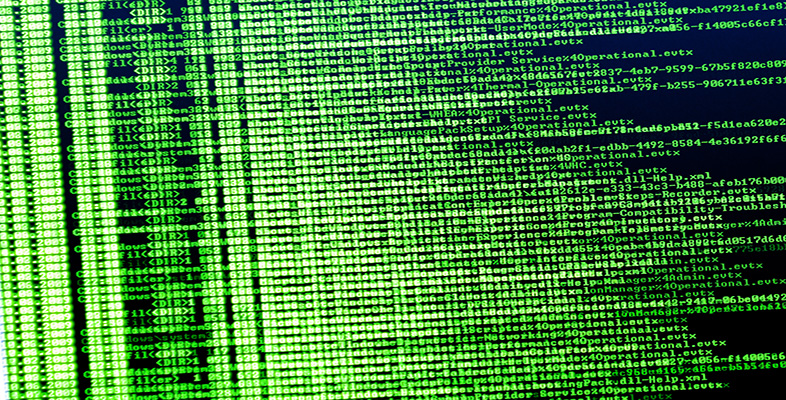Exploring data: Graphs and numerical summaries

Start this free course now. Just create an account and sign in. Enrol and complete the course for a free statement of participation or digital badge if available.

Free course

# 6 Conclusion

In this course, you have been introduced to a number of ways of representing data graphically and of summarizing data numerically. We began by looking at some data sets and considering informally the kinds of questions they might be used to answer.

An important first stage in any assessment of a collection of data, preceding any numerical analysis, is to represent the data, if possible, in some informative diagrammatic way. Useful graphical representations that you have met in this course include pie charts, bar charts, histograms and scatterplots. Pie charts and bar charts are generally used with categorical data, or with numerical data that are discrete (counted rather than measured). Histograms are generally used with continuous (measured) data, and scatterplots are used to investigate the relationship between two numerical variables (which are often continuous but may be discrete). You have seen that a transformation may be useful to aid the representation of data.

However, most diagrammatic representations have some disadvantages. In particular, pie charts are hard to assess unless the data set is simple, with a restricted number of categories. Histograms need a reasonably large data set. They are also sensitive to the choice of cutpoints and the widths of the classes.

Numerical summaries of data are very important. You have been introduced to two main pairs of statistics for assessing location and dispersion. The principal measures of location that have been discussed are the mean and the median, and the principal measures of dispersion are the interquartile range and the standard deviation (together with a related measure, the variance). Because of the way they are calculated, these measures ‘go together’ in pairs – the median with the interquartile range, the mean with the standard deviation. The median and interquartile range are more resistant than are the mean and standard deviation; that is, they are less affected by one or two unusual values in a data set.

The mode has also been introduced. The term ‘mode’ is used for the most frequently occurring value in a set of categorical data, as well as to describe a clear peak in the histogram of a set of continuous data.

You have learned about the terms used to describe lack of symmetry in a data set. A data set is said to be right-skew or positively skewed if a histogram (or bar chart, for numerical discrete data) has a relatively large and long tail towards the higher values, on the right of the diagram. The terms left-skew and negatively skewed are used when there is a relatively long tail towards the lower values, on the left of the diagram. Note that the direction of the tail, and not the direction of the main concentration of the data values, is used to describe the skewness. The sample skewness, which is a numerical summary measure of skewness, has also been defined.

M248_1